# Optical Isomerism Extension RS isomers Written by A

• Slides: 10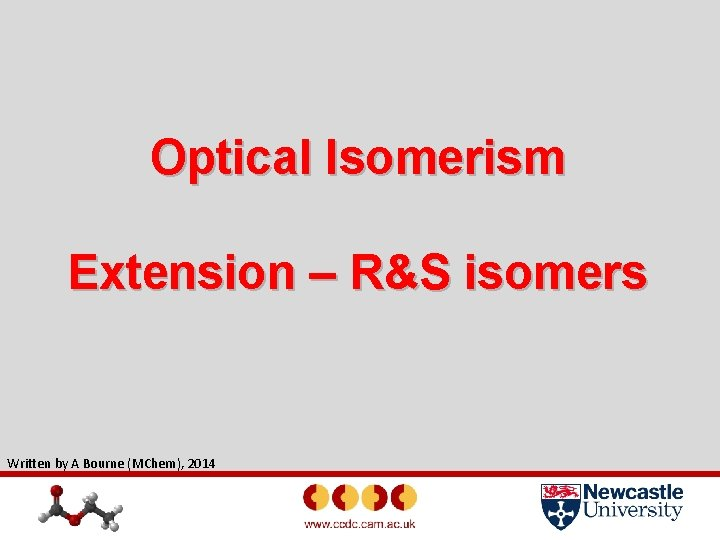Optical Isomerism Extension – R&S isomers Written by A Bourne (MChem), 2014Aims of this Lesson • To assign optical isomers as R or S • Increase your spatial skills and awareness using software designed by the CCDC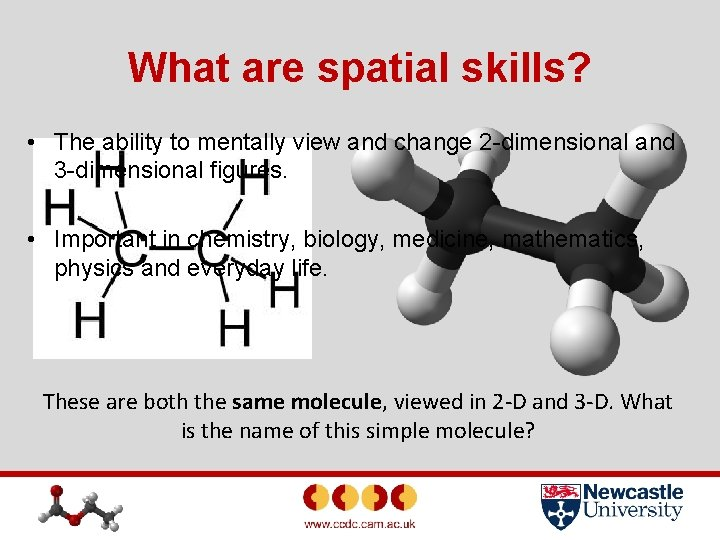What are spatial skills? • The ability to mentally view and change 2 -dimensional and 3 -dimensional figures. • Important in chemistry, biology, medicine, mathematics, physics and everyday life. These are both the same molecule, viewed in 2 -D and 3 -D. What is the name of this simple molecule?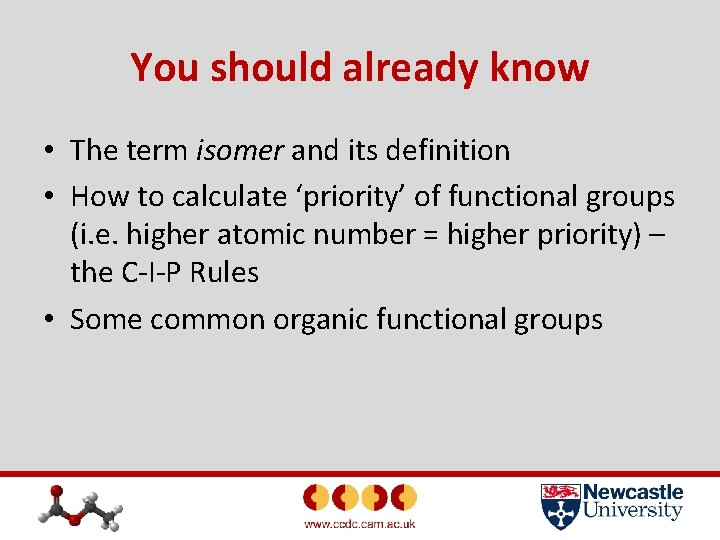You should already know • The term isomer and its definition • How to calculate ‘priority’ of functional groups (i. e. higher atomic number = higher priority) – the C-I-P Rules • Some common organic functional groupsHow do you identify chiral centres? 1. Draw the displayed or skeletal formula of the compound. 2. Check each carbon atom - do any have four different groups bonded? 3. Draw one isomer of the molecule using the ‘wedge-dash’ notation to show the tetrahedral carbon atom. 4. Draw a dotted/dashed line to represent the mirror plane. 5. Draw the mirror image on the other side of the mirror line.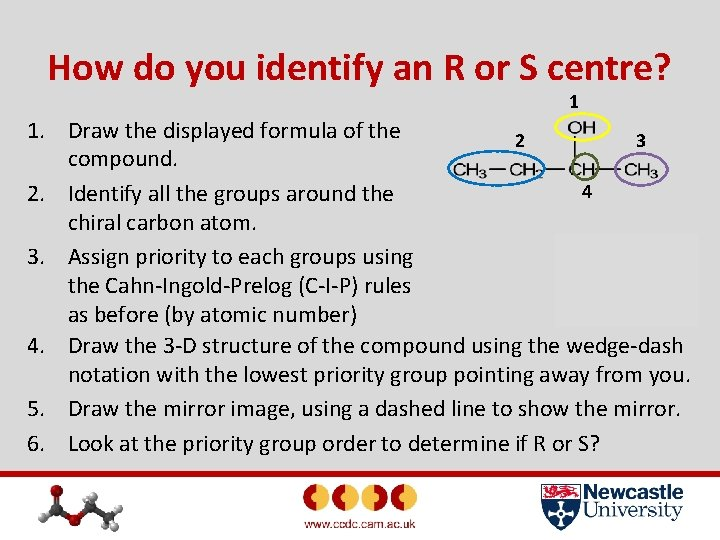How do you identify an R or S centre? 1 1. Draw the displayed formula of the 2 3 compound. 4 2. Identify all the groups around the chiral carbon atom. 3. Assign priority to each groups using the Cahn-Ingold-Prelog (C-I-P) rules as before (by atomic number) 4. Draw the 3 -D structure of the compound using the wedge-dash notation with the lowest priority group pointing away from you. 5. Draw the mirror image, using a dashed line to show the mirror. 6. Look at the priority group order to determine if R or S?Assigning priority - Recap 1. 2. Priority is assigned by atomic number (C-I-P Rules) Only assign by mass number if you have isotopes e. g. 2 H is higher priority than 1 H. With complex groups… 1. 2. 3. Start by assigning priority of each atom adjacent to the chiral carbon. If the atoms are the same, move onto next atoms along. If you have a double bond, count the atom bonded twice.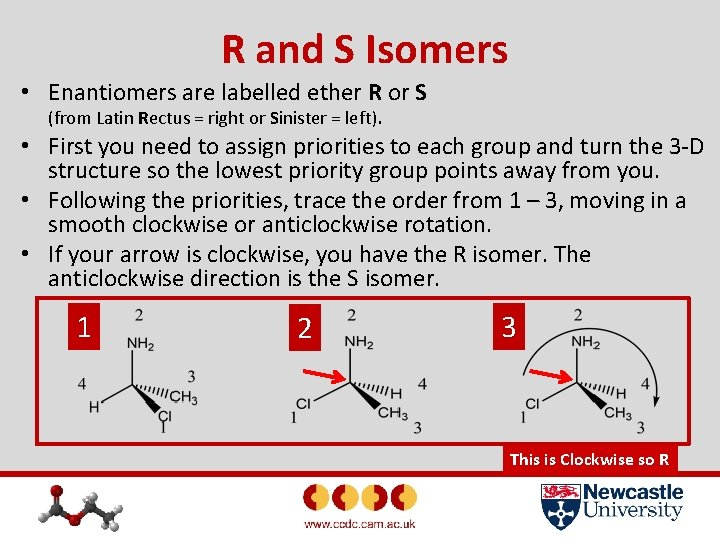R and S Isomers • Enantiomers are labelled ether R or S (from Latin Rectus = right or Sinister = left). • First you need to assign priorities to each group and turn the 3 -D structure so the lowest priority group points away from you. • Following the priorities, trace the order from 1 – 3, moving in a smooth clockwise or anticlockwise rotation. • If your arrow is clockwise, you have the R isomer. The anticlockwise direction is the S isomer. 1 2 3 This is Clockwise so RLet’s have a go…. . Decide if each of these molecules are the R or S isomer. Remember to work through all the steps. 1 2 S 3 R RTask • Do worksheet F 3 – Optical Isomers Extension • If you haven’t used these resources before, you must download and follow sheet A 2 – Introduction to Access Structures first!